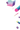# Schedules

Schedules are generators that provide different rates, schedules, decays or series. They’re typically used for batch sizes or learning rates. You can easily implement your own schedules as well: just write your own generator function, that produces whatever series of values you need. A common use case for schedules is within `Optimizer` objects, which accept iterators for most of their parameters. See the training guide for details.

## constant function

Yield a constant rate.``````from thinc.api import constant

batch_sizes = constant(0.001)
batch_size = next(batch_sizes)
``````
```config```[batch_size]
@schedules = "constant.v1"
rate = 0.001
``````
ArgumentType
`rate``float`
YIELDS`float`

## constant_then function

Yield a constant rate for N steps, before starting a schedule.``````from thinc.api import constant_then, decaying

learn_rates = constant_then(
0.005,
1000,
decaying(0.005, 1e-4)
)
learn_rate = next(learn_rates)
``````
```config```[learn_rates]
@schedules = "constant_then.v1"
rate = 0.005
steps = 1000

[learn_rates.schedule]
@schedules = "decaying"
base_rate = 0.005
decay = 1e-4
``````
ArgumentType
`rate``float`
`steps``int`
`schedule``Iterable[float]`
YIELDS`float`

## decaying function

Yield an infinite series of linearly decaying values, following the schedule `base_rate * 1 / (1 + decay * t)`.``````from thinc.api import decaying

learn_rates = decaying(0.005, 1e-4)
learn_rate = next(learn_rates)  # 0.001
learn_rate = next(learn_rates)  # 0.00999
``````
```config```[learn_rate]
@schedules = "decaying.v1"
base_rate = 0.005
decay = 1e-4
t = 0
``````
ArgumentType
`base_rate``float`
`decay``float`
keyword-only
`t``int`
YIELDS`float`

## compounding function

Yield an infinite series of compounding values. Each time the generator is called, a value is produced by multiplying the previous value by the compound rate.``````from thinc.api import compounding

batch_sizes = compounding(1.0, 32.0, 1.001)
batch_size = next(batch_sizes)  # 1.0
batch_size = next(batch_sizes)  # 1.0 * 1.001
``````
```config```[batch_size]
@schedules = "compounding.v1"
start = 1.0
stop = 32.0
compound = 1.001
t = 0
``````
ArgumentType
`start``float`
`stop``float`
`compound``float`
keyword-only
`t``int`
YIELDS`float`

## warmup_linear function

Generate a series, starting from an initial rate, and then with a warmup period, and then a linear decline. Used for learning rates.``````from thinc.api import warmup_linear

learn_rates = warmup_linear(0.01, 3000, 6000)
learn_rate = next(learn_rates)
``````
```config```[learn_rate]
@schedules = "warmup_linear.v1"
initial_rate = 0.01
warmup_steps = 3000
total_steps = 6000
``````
ArgumentType
`initial_rate``float`
`warmup_steps``int`
`total_steps``int`
YIELDS`float`

## slanted_triangular function

Yield an infinite series of values according to Howard and Ruder’s (2018) “slanted triangular learning rate” schedule.``````from thinc.api import slanted_triangular

learn_rates = slanted_triangular(0.1, 5000)
learn_rate = next(learn_rates)
``````
```config```[learn_rate]
@schedules = "slanted_triangular.v1"
max_rate = 0.1
num_steps = 5000
cut_frac = 0.1
ratio = 32
decay = 1.0
t = 0.1
``````
ArgumentType
`max_rate``float`
`num_steps``int`
keyword-only
`cut_frac``float`
`ratio``int`
`decay``float`
`t``float`
YIELDS`float`

## cyclic_triangular function

Linearly increasing then linearly decreasing the rate at each cycle.``````from thinc.api import cyclic_triangular

learn_rates = cyclic_triangular(0.005, 0.001, 1000)
learn_rate = next(learn_rates)
``````
```config```[learn_rate]
@schedules = "cyclic_triangular.v1"
min_lr = 0.005
max_lr = 0.001
period = 1000
``````
ArgumentType
`min_lr``float`
`max_lr``float`
`period``int`
YIELDS`float`